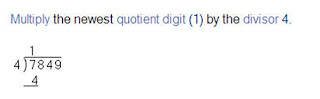## Monday, December 29, 2008

### Math Step-by-Step Help

Struggling with solving your math homework? Sometimes you have most of the problem figured out, but the last step is where you get stuck. Where do you turn to for help especially if you are working on Algebra, Calculus or Trig? Chances are that mom and dad forgot how to do this once they left a formal schooling environment. Try mathaway.com!

Using Mathaway, you can type in your problem ranging from Basic Math through Calculus (no option for Geometry at this time) and Mathaway will lay out how to solve the problem step-by-step. One of my friends was lamenting over Algebra homework - she had one problem left to do and was stuck. I sure don’t remember how to do this. Mathaway came to the rescue! It provided clear step-by-step directions on how to reach the solution.

I was more impressed with Mathaway for higher-level math than for basic math. For example, if I entered a basic math problem that was addition, subtraction or multiplication, Mathaway merely gave me the answer without showing me the steps or the process of how it arrived at the answer. (See below)But if I entered a long division problem, it was beautiful! Everything was written out step by step with clear explanation. (See one step of this problem below.) Mathaway had similar results for the higher level math problems, so I can see where this site would be a life-saver.Overall, I like Mathaway for higher-level math problems and not for basic math. I think this could be the key to helping students who are missing one step in a process, and don’t have anyone at home who can help them.